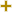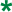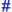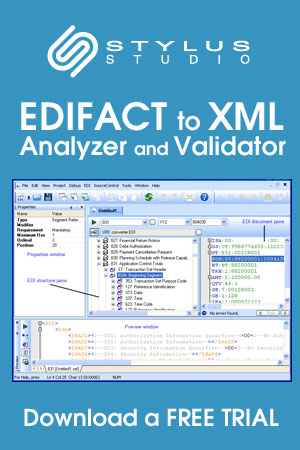# Date/time/period format qualifierUN/CEFACT Revision 1995A Code List

Repr:an..3
Desc:Specification of the representation of a date, a date and time or of a period.
This table also occurs in the following versions of this standard:
D00A, D00B, D01A, D01B, D01C, D02A, D02B, D03A, D03B, D04A, D04B, D93A, D94A, D94B, D95A, D95B, D96A, D96B, D97A, D97B, D98A, D98B, D99A, D99B

2DDMMYY
Calendar date: D = Day; M = Month; Y = Year.
3MMDDYY
Calendar date: M = Month; D = Day; Y = Year.
101YYMMDD
Calendar date: Y = Year; M = Month; D = Day.
102CCYYMMDD
Calendar date: C = Century ; Y = Year ; M = Month ; D = Day.
103YYWWD
Calendar week day: Y = Year ; W = Week ; D = Day Week number 01 is always first week of January Day number 1 is always Monday.
105YYDDD
Calendar day: Y = Year ; D = Day January the first = Day 001 Always start numbering the days of the year from January 1st through December 31st.
106MMDD
Day of a month: M = Month; D = Day.
107DDD
Day's number within a specific year: D = Day.
108WW
Week's number within a specific year: W = Week.
109MM
Month's number within a specific year: M = Month.
110DD
Day's number within is a specific month: D = Day.
201YYMMDDHHMM
Calendar date including time without seconds: Y = Year; M = Month; D = Day; H = Hour; M = Minute.
202YYMMDDHHMMSS
Calendar date including time with seconds: Y = Year; M = Month; D = Day; H = Hour; m = Minutes = Seconds.
203CCYYMMDDHHMM
Calendar date including time with minutes: C=Century; Y=Year; M=Month; D=Day; H=Hour; M=Minutes.
204CCYYMMDDHHMMSS
Calendar date including time with seconds: C=Century;Y=Year; M=Month;D=Day;H=Hour;M=Minute;S=Second.
301YYMMDDHHMMZZZ
See 201 + Z = Time zone.
302YYMMDDHHMMSSZZZ
See 202 + Z = Time zone.
303CCYYMMDDHHMMZZZ
See 203 plus Z=Time zone.
304CCYYMMDDHHMMSSZZZ
See 204 plus Z=Time zone.
305MMDDHHMM
Month, day, hours, minutes; M = Month; D = Day; H = Hour; M = Minute.
306DDHHMM
Day, hours, minutes; D = Day; H = Hour; M = Minute.
401HHMM
Time without seconds: H = Hour; m = Minute.
402HHMMSS
Time with seconds: H = Hour; m = Minute; s = Seconds.
404HHMMSSZZZ
See 402 plus Z=Time zone.405
MMMMSS
Time without hours: m=minutes, s=seconds.
501HHMMHHMM
Time span without seconds: H = Hour; m = Minute;.
502HHMMSS-HHMMSS
Format of period to be given without hyphen.
503HHMMSSZZZ-HHMMSSZZZ
Format of period to be given without hyphen.
600CC
Century.
601YY
Calendar year: Y = Year.
602CCYY
Calendar year including century: C = Century; Y = Year.
603YYS
Semester in a calendar year: Y = Year; S = Semester.
604CCYYS
Semester in a calendar year: C = Century; Y = Year; S = Semester.
608CCYYQ
Quarter in a calendar year: C = Century; Y = Year; Q = Quarter.
609YYMM
Month within a calendar year: Y = Year; M = Month.
610CCYYMM
Month within a calendar year: CC = Century; Y = Year; M = Month.
613YYMMA
Format of period to be given without hyphen (A = ten days period).
614CCYYMMA
Format of period to be given without hyphen (A = ten days period).
615YYWW
Week within a calendar year: Y = Year; W = Week 1st week of january = week 01.
616CCYYWW
Week within a calendar year: CC = Century; Y = Year; W = Week (1st week of January = week 01).
701YY-YY
Format of period to be given in actual message without hyphen.
702CCYY-CCYY
Format of period to be given in actual message without hyphen.
703YYS-YYS
Format of period to be given without hyphen.
704CCYYS-CCYYS
Format of period to be given in actual message without hyphen.
705YYPYYP
Format of period to be given without hyphen (P = period of 4 months).
706CCYYP-CCYYP
Format of period to be given without hyphen (P = period of 4 months).
707YYQ-YYQ
Format of period to be given without hyphen.
708CCYYQ-CCYYQ
Format of period to be given in actual message without hyphen.
709YYMM-YYMM
Format of period to be given in actual message without hyphen.
710CCYYMM-CCYYMM
Format of period to be given in actual message without hyphen.
711CCYYMMDD-CCYYMMDD
Format of period to be given in actual message without hyphen.
713YYMMDDHHMM-YYMMDDHHMM
Format of period to be given in actual message without hyphen.
715YYWW-YYWW
Format of period to be given in actual message without hyphen.
716CCYYWW-CCYYWW
Format of period to be given without hyphen.
717YYMMDD-YYMMDD
Format of period to be given in actual message without hyphen.
718CCYYMMDD-CCYYMMDD
Format of period to be given without hyphen.
801Year
To indicate a quantity of years.
802Month
To indicate a quantity of months.
803Week
To indicate a quantity of weeks.
804Day
To indicate a quantity of days.
805Hour
To indicate a quantity of hours.
806Minute
To indicate a quantity of minutes.
807Second
To indicate a quantity of seconds.
808Semester
To indicate a quantity of semesters (six months).
809Four months period
To indicate a quantity of four months periods.
810Trimester
To indicate a quantity of trimesters (three months).
811Half month
To indicate a quantity of half months.
812Ten days
To indicate a quantity of ten days periods.
813Day of the week
Numeric representation of the day (Monday = 1).814
Working days
Number of working days.

Change indicators
plus signAn addition.
asteriskAddition/substraction/change to a code entry for a particular data element.
hash or pound signChanges to names.
vertical barChanges to text for descriptions, notes and functions.
minus signA deletion.
letter XMarked for deletion.